### Home > A2C > Chapter 9 > Lesson 9.3.3 > Problem9-175

9-175.
1. The roots of two quadratic polynomials are given below. Write possible quadratic functions in standard form. Homework Help ✎

1.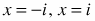2.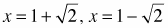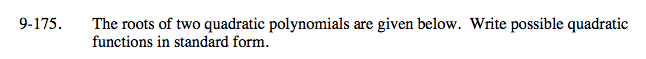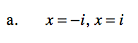(x + i)(xi) = x2 + 1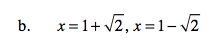$(\textit{x}-(1+\sqrt{2}))(\textit{x}-(1-\sqrt{2}))=?$